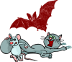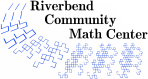# Catz, Ratz, and Batz

### Description

Students learn to play a dice game called Catz, Ratz, and Batz. Analysis of the game reveals a quadratic function that governs the maximum possible score on each turn. Students also consider questions of expected value corresponding to various choices in the game to determine what is the best option.

### Levels

• This activity can be extended to an undergraduate research project.

5 minutes

### Activity Time

60 to 120 minutes

### Topics

• multiplication facts
• modeling
• probability
• expected value

### Goals

• Students will explore a scenario that can be modeled by a quadratic function.
• Students will compute expected values to discover the best strategy for certain situations in a game.

### Materials

• Notebook Paper
• Graph Paper
• Pencils
• Catz, Ratz, and Batz games for groups to play

### Prerequisites

• Multiplication
• Familiarity with expected value and probability concepts

### Credits

Catz, Ratz, and Batz. Created by Maureen Hiron and published by Playroom Entertainment in 2003.

### Author

Amanda Katharine Serenevy

### National Common Core Standards

 HS.F-IF.4 For a function that models a relationship between two quantities, interpret key features of graphs and tables in terms of the quantities, and sketch graphs showing key features given a verbal description of the relationship. Key features include intercepts, increasing/decreasing intervals, positive/negative values, maxima/minima, symmetries, end behavior, and periodicity. HS.Modeling Modeling is the process of choosing and using appropriate mathematics and statistics to analyze empirical situations, to understand them better, and to improve decisions. Quantities and their relationships in physical, economic, public policy, social, and everyday situations can be modeled using mathematical and statistical methods. When making mathematical models, technology is valuable for varying assumptions, exploring consequences, and comparing predictions with data. HS.S-CP.6 Find the conditional probability of A given B as the fraction of B's outcomes that also belong to A, and interpret the answer in terms of the model. HS.S-MD.5 Weigh the possible outcomes of a decision by assigning probabilities to payoff values and finding expected values.
Riverbend Community Math Center
hello@riverbendmath.org
http://riverbendmath.org
(574) 339-9111This work placed into the public domain by the Riverbend Community Math Center.